output.to from Sideway
Number Theory

Factorization

Pollard's p-1 Method

Draft for Information Only

# Content

``` Pollard's p-1 Method     Characteristic of Pollard's p-1 Method by Smooth Number     Pollard's P-1 Methed by Smooth Number Example 3```

# Pollard's p-1 Method

Pollard's p-1 method is a prime factorization algorithm discovered by John Pollard in 1974. Limited by the algorithm, the Pollard's p-1 method is only work for integers with specific factors.

However, since the composite number n is an unknown, sometimes, the algorithm may return a false response.

#### Characteristic of Pollard's p-1 Method by Smooth Number

The advantage of Pollard's p-1 method by smooth number is the checking of a group of  primes with one computation. Every boundary B represents a group of numbers that can be expressed as  the product of primes less than and equal to number B.

For example, a composite number n less than 10000 should have a prime factor less than √n=100. And primes within 100 are

Primes Integer B-smooth count
2, 3, 5, 7, 11, 13, 17, 19, 23, 29, 31, 37, 41, 43, 47, 53, 59, 61, 67, 71, 73, 79, 83, 89, 97 p   25
2, 3, 5, 17 p-1 2
2, 3, 5, 7, 13, 17, 19, 37, 73, 97 p-1 3 10
2, 3, 5, 7, 11, 13, 17, 19, 31, 37, 41, 61, 73, 97 p-1 5 14
2, 3, 5, 7, 11, 13, 17, 19, 29, 31, 37, 41, 43, 61, 71, 73, 97 p-1 7 17
2, 3, 5, 7, 11, 13, 17, 19, 23, 29, 31, 37, 41, 43, 61, 67, 71, 73, 89, 97 p-1 11 20
2, 3, 5, 7, 11, 13, 17, 19, 23, 29, 31, 37, 41, 43, 53, 61, 67, 71, 73, 79, 89, 97 p-1 13 22
2, 3, 5, 7, 11, 13, 17, 19, 23, 29, 31, 37, 41, 43, 53, 61, 67, 71, 73, 79, 89, 97 p-1 17 22
2, 3, 5, 7, 11, 13, 17, 19, 23, 29, 31, 37, 41, 43, 53, 61, 67, 71, 73, 79, 89, 97 p-1 19 22
2, 3, 5, 7, 11, 13, 17, 19, 23, 29, 31, 37, 41, 43, 47, 53, 61, 67, 71, 73, 79, 89, 97 p-1 23 23
2, 3, 5, 7, 11, 13, 17, 19, 23, 29, 31, 37, 41, 43, 47, 53, 59, 61, 67, 71, 73, 79, 89, 97 p-1 29 24
2, 3, 5, 7, 11, 13, 17, 19, 23, 29, 31, 37, 41, 43, 47, 53, 59, 61, 67, 71, 73, 79, 89, 97 p-1 31 24
2, 3, 5, 7, 11, 13, 17, 19, 23, 29, 31, 37, 41, 43, 47, 53, 59, 61, 67, 71, 73, 79, 89, 97 p-1 37 24
2, 3, 5, 7, 11, 13, 17, 19, 23, 29, 31, 37, 41, 43, 47, 53, 59, 61, 67, 71, 73, 79, 83, 89, 97 p-1 41 25
2, 3, 5, 7, 11, 13, 17, 19, 23, 29, 31, 37, 41, 43, 47, 53, 59, 61, 67, 71, 73, 79, 83, 89, 97 p-1 43 25
2, 3, 5, 7, 11, 13, 17, 19, 23, 29, 31, 37, 41, 43, 47, 53, 59, 61, 67, 71, 73, 79, 83, 89, 97 p-1 47 25

More primes can be included for checking by increasing the smoothness boundary B.

For checking n and ak-1 is divisible by p, k is selected sufficiently large to ensure p-1 divides k, If the specific type prime factor is  less than กิn, in the worst case, the smoothness boundary B can be equal to 41.

However, since the composite number n is an unknown, sometimes, the algorithm may return a false response. For example:

##### Pollard's P-1 Methed by Smooth Number Example 3

For example: n=533=p*q=13*41; let B=5 imply

Integer B-smooth number Prime Factors number
k 5 29*35*53 = 512*243*125 15552000

Therefore for B=5, k5 or (p5-1)m5 is equal to 15552000.

###### Fermat's Little Theorem

let a=2, by Fermat's little theorem, let p be one of the prime factors of n, imply p divides ak-1.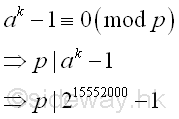###### Greatest Common Divisor

Since ak-1 is a very large number, before finding the greatest common divisor of n and ak-1,  ak-1 can be raised to the high power modulo n. Imply

Using squarings modulo

base  number; a=2; k=15552000; n=533
ak base 10 215552000
ai base 10 21  = 21 ก 2 (mod 533)
22 = 22 ≡ 4 (mod 533)
24 = 42 ≡ 16 (mod 533)
28 = 162 ≡ 256 (mod 533)
216 = 2562 ≡ 510 (mod 533)
232 = 5102 ≡ 529 (mod 533)
264 = 5292 ≡ 16 (mod 533)
2128 = 162 ≡ 256 (mod 533)
2256 = 2562 ≡ 510 (mod 533)
2512 = 5102 ≡ 529 (mod 533)
21024 = 5292 ≡ 16 (mod 533)
22048 = 162 ≡ 256 (mod 533)
24096 = 2562 ≡ 510 (mod 533)
28192 = 5102 ≡ 529 (mod 533)
216384 = 5292 ≡ 16 (mod 533)
232768 = 162 ≡ 256 (mod 533)
265536 = 2562 ≡ 510 (mod 533)
2131072 = 5102 ≡ 529 (mod 533)
2262144 = 5292 ≡ 16 (mod 533)
2524288 = 162 ≡ 256 (mod 533)
21048576 = 2562 ≡ 510 (mod 533)
22097152 = 5102 ≡ 529 (mod 533)
24194304 = 5292 ≡ 16 (mod 533)
28388608 = 162 ≡ 256 (mod 533)
216777216 = 5092 ≡ 285 (mod 533)
233554432 = 2852 ≡ 518 (mod 533)
267108864 = 5182 ≡ 190 (mod 533)
ak base 10 28388608+4194304+2097152+524288+262144+65536+16384+2048+1024+512
ak base 10 28388608*24194304*22097152*2524288*2262144* 265536*216384*22048*21024*2512
ak base 10 256*16*529*256*16*510*16*256*16*529 ≡ 1 (mod 533)

Imply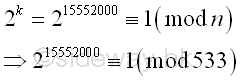The algorithm returns a fail response, because the number n divides ak-1 and n is the greatest common divisor of n and ak-1. Imply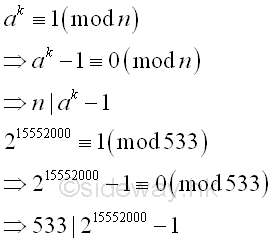Therefore every prime factor of number n divides ak-1, imply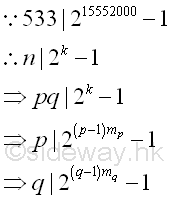Since k is a very large number, usually the algorithm fails because k is the multiple of the product of p-1 and q-1, imply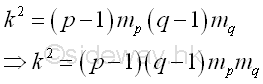One way is to select a smaller k so that k is not the common multiple of both p-1 and q-1. Let B=3, Imply

Integer B-smooth number Prime Factors number
k 3 29*35 = 512*243 124416

Therefore for B=3, k3 or (p3-1)m3 is equal to 124416.

###### Fermat's Little Theorem

let a=2, by Fermat's little theorem, let p be one of the prime factors of n, imply p divides ak-1.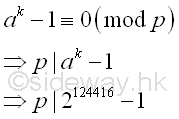###### Greatest Common Divisor

Since ak-1 is a very large number, before finding the greatest common divisor of n and ak-1,  ak-1 can be raised to the high power modulo n. Imply

Using squarings modulo

base  number; a=2; k=124416; n=533
ak base 10 2124416
ai base 10 21  = 21 ก 2 (mod 533)
22 = 22 ≡ 4 (mod 533)
24 = 42 ≡ 16 (mod 533)
28 = 162 ≡ 256 (mod 533)
216 = 2562 ≡ 510 (mod 533)
232 = 5102 ≡ 529 (mod 533)
264 = 5292 ≡ 16 (mod 533)
2128 = 162 ≡ 256 (mod 533)
2256 = 2562 ≡ 510 (mod 533)
2512 = 5102 ≡ 529 (mod 533)
21024 = 5292 ≡ 16 (mod 533)
22048 = 162 ≡ 256 (mod 533)
24096 = 2562 ≡ 510 (mod 533)
28192 = 5102 ≡ 529 (mod 533)
216384 = 5292 ≡ 16 (mod 533)
232768 = 162 ≡ 256 (mod 533)
265536 = 2562 ≡ 510 (mod 533)
ak base 10 265536+32768+16384+8192+1024+512
ak base 10 265536*232768*216384*28192*21024*2512
ak base 10 510*256*16*529*16*529 ≡ 469 (mod 533)

Imply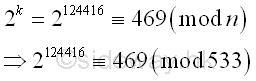The greatest common divisor of n and ak-1 is

Using Euclid's algorithm

ak-1 n
2124416-1 533
469-1 533
468 533-468=65
468-7*65=13 65
13 65-5*13=0
13 0

Imply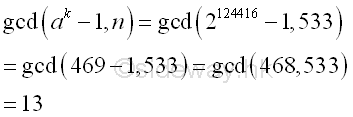Integer 13, the greatest common divisor of n and ak-1 is also the prime divisor of n. And p-1 is  3-smooth.

Integer B-smooth number Prime Factors number
p-1 3 22*31 12
k 3 29*35 = 512*243 124416
k/(p-1)   27*34 10368

Besides, for B=5

Integer B-smooth number Prime Factors number
p-1 3,5 22*31 12
q-1 5 23*51 40
k5 5 29*35*53 = 512*243*125 15552000
k5/(p-1)(q-1)   24*34*52 32400

ID: 120500008 Last Updated: 16/5/2012 Revision: 0Home 5

Management

HBR 3

Information

Recreation

Culture

Chinese 1097

English 337

Computer

Hardware 149

Software

Application 187

Numeric 19

Programming

Web 757

CSS 1

HTML

Knowledge Base

OS 389

MS Windows

Knowledge

Mathematics

Algebra 20

Number Theory 206

Geometry 18

Calculus 67

Engineering

Mechanical

Rigid Bodies

Statics 92

Dynamics 37

Control

Physics

Electric 10